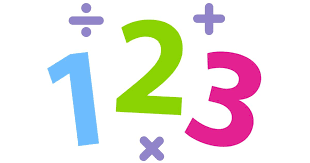# Numeracy

WHAT DO WE LEARN IN MATHEMATICS?

Year Two

Dear Parents and Carers,

Over the coming weeks I will continue to outline what the teaching and learning Mathematics curriculum encompasses for each year level. In this article I will focus on Year Two. In the classroom children may be working at level, below level or above level. Teachers differentiate the teaching and tasks to cater for the learning each child needs to access.

In level 2, students use grouping partitioning and re-arrangement to apply place value and extend the range of numbers they use and apply to thousands. There are three major areas Number and Algebra, Measurement and Geometry, Statistics and Probability.

Number and Algebra

Number and place value

• Investigate number sequences, initially those increasing and decreasing by twos, threes, fives and ten from any starting point, then moving to other sequences
• Recognise, model, represent and order numbers to at least 1000
• Group, partition and rearrange collections up to 1000 in hundreds, tens and ones to facilitate more efficient counting
• Explore the connection between addition and subtraction
• Solve simple addition and subtraction problems using a range of efficient mental and written strategies
• Recognise and represent multiplication as repeated addition, groups and arrays
• Recognise and represent division as grouping into equal sets and solve simple problems using these representations.

Money and financial mathematics

• Count and order small collections of Australian coins and notes according to their value.

Fractions and Decimals

• Recognise and interpret common uses of halves, quarters and eighths of shapes and collections.

Patterns and algebra

• Describe patterns with numbers and identify missing elements
• Solve problems by using number sentences for addition or subtraction
• Apply repetition in arithmetic operations, including multiplication as repeated addition and division as repeated subtraction.

Measurement and Geometry

Using units of measurement

• Compare and order several shapes and objects based on length, area, volume and capacity using appropriate uniform informal units Compare masses of objects using balance scales
• Tell time to the quarter-hour, using the language of 'past' and to
• Name and order months and seasons
• Use a calendar to identify the date and determine the number of days in each month.

Shape

• Describe and draw two-dimensional shapes, with and without digital technologies
• Describe the features of three-dimensional objects

Location and transformation

• Interpret simple maps of familiar locations and identify the relative positions of key features
• Investigate the effect of one-step slides and flips with and without digital technologies
• Identify and describe half and quarter turns.

Statistics and Probability

Chance

• Identify practical activities and everyday events that involve chance.
• Describe outcomes as ‘likely’ or ‘unlikely’ and identify some events as ‘certain’ or ‘impossible.’

Data representation and interpretation

• Identify a question of interest based on one categorical variable. Gather data relevant to the question
• Collect, check and classify data
• Create displays of data using lists, table and picture graphs and interpret them.